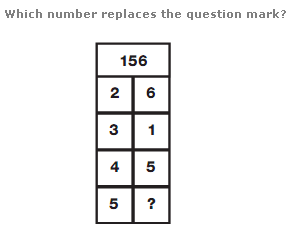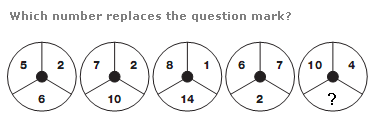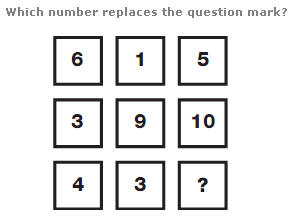# Puzzles - Number puzzles

Exercise : Number puzzles
208.:
4
Explanation
:
Reading each pair of numbers as 2 digit values, the sum of the 4 numbers is given in the top space in the diagram.

209.:
12
Explanation
:
In each circle, the number in the lower segment equals twice the difference between the numbers in the left and right hand segments.

210.